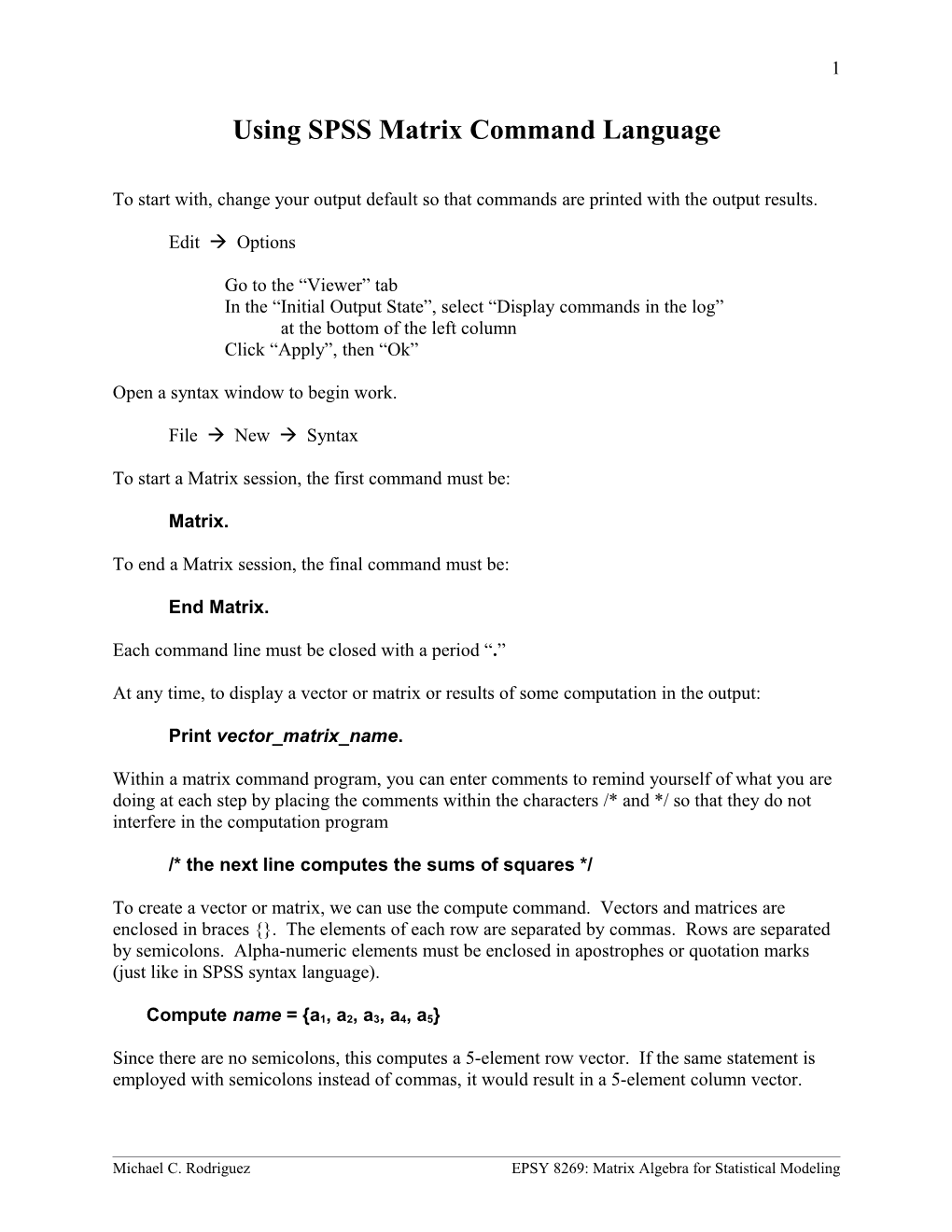# Using SPSS Matrix Command Language1

Using SPSS Matrix Command Language

Edit  Options

Go to the “Viewer” tab

In the “Initial Output State”, select “Display commands in the log”

at the bottom of the left column

Click “Apply”, then “Ok”

Open a syntax window to begin work.

File  New  Syntax

To start a Matrix session, the first command must be:

Matrix.

To end a Matrix session, the final command must be:

End Matrix.

Each command line must be closed with a period “.”

At any time, to display a vector or matrix or results of some computation in the output:

Print vector_matrix_name.

Within a matrix command program, you can enter comments to remind yourself of what you are doing at each step by placing the comments within the characters /* and */ so that they do not interfere in the computation program

/* the next line computes the sums of squares */

To create a vector or matrix, we can use the compute command. Vectors and matrices are enclosed in braces {}. The elements of each row are separated by commas. Rows are separated by semicolons. Alpha-numeric elements must be enclosed in apostrophes or quotation marks (just like in SPSS syntax language).

## Compute name = {a1, a2, a3, a4, a5}

Since there are no semicolons, this computes a 5-element row vector. If the same statement is employed with semicolons instead of commas, it would result in a 5-element column vector.

A simple set of commands that creates a 5-element row vector and a column vector is:

Matrix.

compute x = {1,3,2,4,3}.

print x.

compute y = {3;2;4;3;5}.

print y.

End Matrix.

Run MATRIX procedure:

X

1 3 2 4 3

Y

3

2

4

3

5

------END MATRIX -----

To maintain agreement with notation used in class, we will engage SPSS Matrix command language in a way that only employs column vectors and consider row vectors to be the Transpose of the column vector.

The command to Transpose a column vector is:

Compute a_prime = T(a).

We can now employ basic arithmetic operations on vectors using arithmetic operators.

Compute k = a + b.

When necessary, you can make a vector or matrix where all elements are the same value as specified in the command. This is useful for creating ones vectors and null vectors.

Compute ones = Make(5,1,1).

This Make command is in general form Make (S1, S2, S3) where S1 is the number of rows, S2 is the number of columns, and S3 is the specific value for each element.

MATRIX.

compute a = {3;7;5;11;4;0}.

compute b = {4;-2;2;6;-4;0}.

compute c = {0;-7;.5;6;6;6}.

compute e = {5;0;2;2;3}.

compute lambda = {-1}.

print a.

print b.

print c.

print e.

print lambda.

Run MATRIX procedure:

A

3

7

5

11

4

0

B

4

-2

2

6

-4

0

C

.000000000

-7.000000000

.500000000

6.000000000

6.000000000

6.000000000

E

5

0

2

2

3

LAMBDA

-1

compute te = T(e).

print te.

TE

5 0 2 2 3

compute m = a + b.

print m.

M

7

5

7

17

0

0

compute n = T(b) - T(c).

print n.

N

Columns 1 - 5

4.00000000 5.00000000 1.50000000 .00000000 -10.00000000

Columns 6 - 6

-6.00000000

Michael C. RodriguezEPSY 8269: Matrix Algebra for Statistical Modeling

1

Michael C. RodriguezEPSY 8269: Matrix Algebra for Statistical Modeling

1

compute q = lambda*b.

print q.

Q

-4

2

-2

-6

4

0

compute u = lambda*T(e).

print u.

U

-5 0 -2 -2 -3

compute w = T(a)*b.

print w.

W

58

compute ss = T(e)*e.

print ss.

SS

42

compute ones = Make(5,1,1).

print ones.

ONES

1

1

1

1

1

compute x = T(e)*ones.

print x.

X

12

compute y = T(ones)*e.

print y.

Y

12

End Matrix.

Michael C. RodriguezEPSY 8269: Matrix Algebra for Statistical Modeling